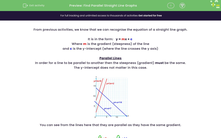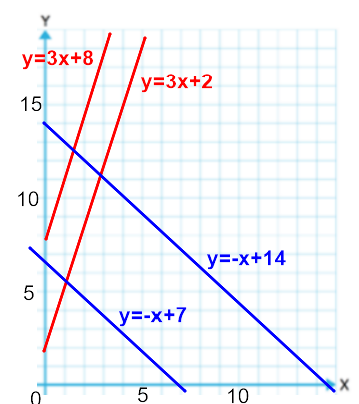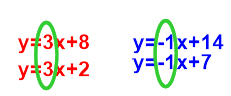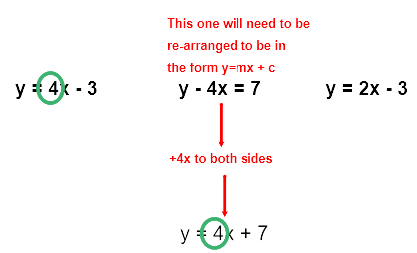# Find Parallel Straight Line Graphs

In this worksheet, students will use an equation for a straight line graph to recognise parallel linesKey stage:  KS 3

Curriculum topic:   Algebra

Curriculum subtopic:   Use Linear Equations (Two Variables) and Gradients

Difficulty level:#### Worksheet Overview

From previous activities, we know that we can recognise the equation of a straight line graph.

It is in the form:   y = mx +

Where m is the gradient (steepness) of the line

and c is the y-intercept (where the line crosses the y axis)

Parallel Lines

In order for a line to be parallel to another then the steepness (gradient) must be the same.

The y-intercept does not matter in this case.You can see from the lines here that they are parallel as they have the same gradient.This activity is about recognising lines that are parallel to each other.

Example

Which of these lines are parallel?

y = 4x - 3               y - 4x = 7            y = 2x - 3

To be parallel the lines have to have the same gradient.In this case, once they are all in the same form, then the first two have a gradient of 4 so are parallel.

Let's try some questions - remember they all have to be in this form:  y = mx + c### What is EdPlace?

We're your National Curriculum aligned online education content provider helping each child succeed in English, maths and science from year 1 to GCSE. With an EdPlace account you’ll be able to track and measure progress, helping each child achieve their best. We build confidence and attainment by personalising each child’s learning at a level that suits them.

Get started